>

Chapter 14 Quadrilaterals R.D. Sharma Solutions for Class 9th Exercise 14.4

Exercise 14.4

1. In a ΔABC, D, E and F are, respectively, the mid-points of BC, CA and AB. If the lengths of side AB, BC and CA are 7 cm, 8 cm and 9 cm, respectively, find the perimeter of ΔDEF.

SolutionGiven that,
AB = 7cm, BC = 8 cm, AC = 9cm
In △ABC,
F and E are the mid points AB and AC.
∴ EF = 1/2 BC
Similarly,
DF = 1/2 AC and DE = 1/2 AB
Perimeter of △DEF = DE + EF + DF
= 1/2 AB + 1/2 BC + 1/2 AC
=  1/2×7 + 1/2×8 + 1/2×9
= 3.5 + 4 + 4.5 = 12 cm
Perimeter of △DEF = 12 cm.

2. In a triangle ∠ABC, ∠A = 50°, ∠B = 60° and C =∠70°. Find the measures of the angles of the triangle formed by joining the mid-points of the sides of this triangle.

SolutionIn △ABC,
D and E are mid points of AB and BC
By mid-point theorem,
DE∥AC, DE = 1/2 AC
F is the midpoint of AC.
Then, DE = 1/2 AC = CF
DE∥AC, DE = CF
Hence, DECF is a parallelogram.
∠C = ∠D = 70°  [opposites sides of parallelogram]
Similarly,
BEFD is a parallelogram, ∠B = ∠F = 60°
ADEF is a parallelogram, ∠A = ∠E = 50°
∴ Angles of △DEF are ∠D = 70°, ∠E = 50°, ∠F = 60°

3. In a triangle, P, Q and R are the mid-points of sides BC, CA and AB respectively. If AC = 21 cm, BC = 29 cm and AB = 30 cm, find the perimeter of the quadrilateral ARPQ.

Solution

In △ABC,

R and P are mid points of AB and BC
RP∥AC, RP = 1/2 AC  [By midpoint Theorem]
In a quadrilateral, a pair of side is parallel and equal.
RP∥AQ, RP = AQ
Therefore, RPQA is a parallelogram.
⇒ AR = 1/2 AB = 1/2×30 = 15 cm
AR =QP = 15 cm  [Opposites sides are equal]
⇒ RP = 1/2 AC = 1/2×21 = 10.5 cm
RP = AQ = 10.5 cm
Now,
Perimeter of ARPQ = AR + QP + RP + AQ
= 15+15+10.5+10.5 = 51 cm

4. In a ΔABC median AD is produced to X such that AD = DX. Prove that ABXC is a parallelogram

Solution

△ABC is given with AD as the median extended to point X such that AD = DX.Join BX and CX.

We get a quadrilateral ABXC, we need to prove that it’s a parallelogram.
We know that AD is the median.
By definition of median we get:
BD = CD
Also, it is given that
Thus, the diagonals of the quadrilateral ABCX bisect each other.
Therefore, quadrilateral ABXC is a parallelogram.
Hence proved.

5. In a ABC, E and F are the mid-points of AC and AB respectively. The altitude AP to BC intersects FE at Q. Prove that AQ = QP.

Solution

ΔABC is given with E and F as the mid points of sides AB and AC.

Also, AP ⊥BC intersecting EF at Q.
We need to prove that AQ = QP
In △ABC, E and are the mid-points of AB and AC respectively.
Theorem states, the line segment joining the mid-points of any two sides of a triangle is parallel to the third side and equal to half of it.
Therefore, we get: EF ⊥BC
Since, Q lies on EF.
Therefore, FQ | | BC
This means,
Q is the mid-point of AP.
Thus, AQ = QP (Because, F is the mid point of AC and FQ | | BC )
Hence proved.

6. In a ΔABC, BM and CN are perpendiculars from B and C respectively on any line passing through A. If L is the mid-point of BC, prove that ML = NL.

Solution7. In Fig. 14.95, triangle ABC is right-angled at B. Given that AB = 9 cm, AC = 15 cm and D, E are the mid-points of the sides AB and AC respectively, calculate

(i) The length of BC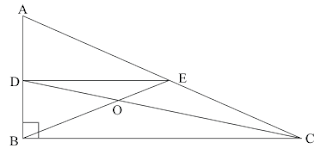Solution8. In Fig. 14.96, M, N and P are the mid-points of AB, AC and BC respectively. If MN = 3 cm, NP = 3.5 cm and MP = 2.5 cm, calculate BC, AB and AC.Solution

Given,

MN = 3cm, NP = 3.5cm and MP = 2.5cm
To find BC, AB and AC
In △ABC,
M and N are mid-points of AB and AC.
∴ MN = 1/2 BC, MN∥BC  [By midpoint theorem]
⇒ 3 = 1/2 BC
⇒ 3×2 = BC
⇒ BC = 6 cm
Similarly,
AC = 2MP = 2×2.5 = 5 cm
AB = 2NP = 2×3.5 = 7 cm

9. ABC is a triangle and through A, B, C lines are drawn parallel to BC, CA and AB respectively intersecting at P, Q and R. Prove that the perimeter of ΔPQR is double the perimeter of ΔABC

Solution10. In Fig. 14.97, BE ⊥ AC. AD is any line from A to BC intersecting BE in H. P, Q and R are respectively the mid-points of AH, AB and BC. Prove that ∠PQR = 90°Solution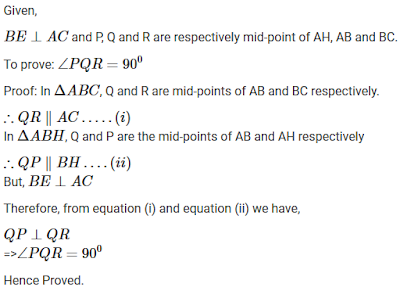11. In Fig. 14.98, AB = AC and CP || BA and AP is the bisector of exterior ∠CAD of ΔABC. Prove that (i) ∠PAC = ∠BCA (ii) ABCP is a parallelogram.Solution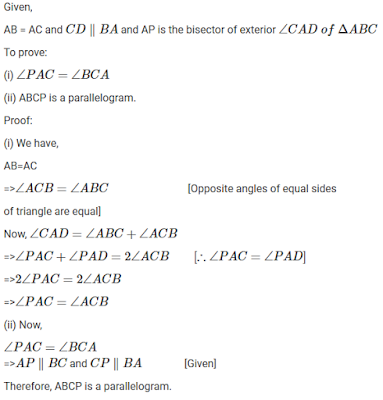12. ABCD is a kite having AB = AD and BC = CD. Prove that the figure formed by joining the mid-points of the sides, in order, is a rectangle.

Solution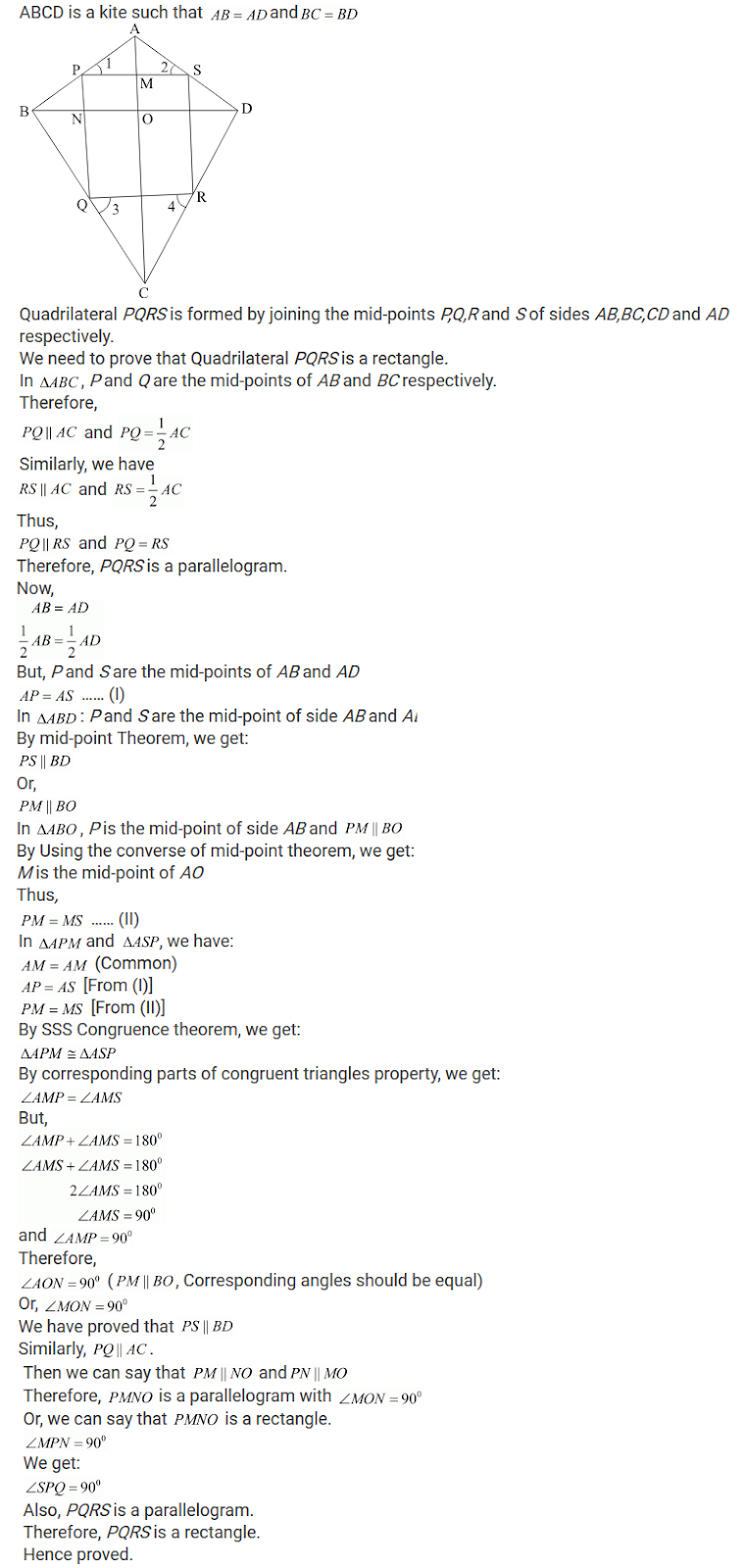13. Let ABC be an isosceles triangle in which AB = AC. If D, E, F be the mid-points of the sides BC, CA and AB respectively, show that the segment AD and EF bisect each other at right angles.

Solution14. ABC is a triangle, D is a point on AB such that AD = 1/4 AB and E is a point on AC such that AE = 1/4 AC. Prove that DE = 1/2 BC.

Solution15. In Fig. 14.99, ABCD is a parallelogram in which P is the mid-point of DC and Q is a point on AC such that CQ=1/4 AC. If PQ produced meets BC at R, Prove that R is a midpoint of BC.

Solution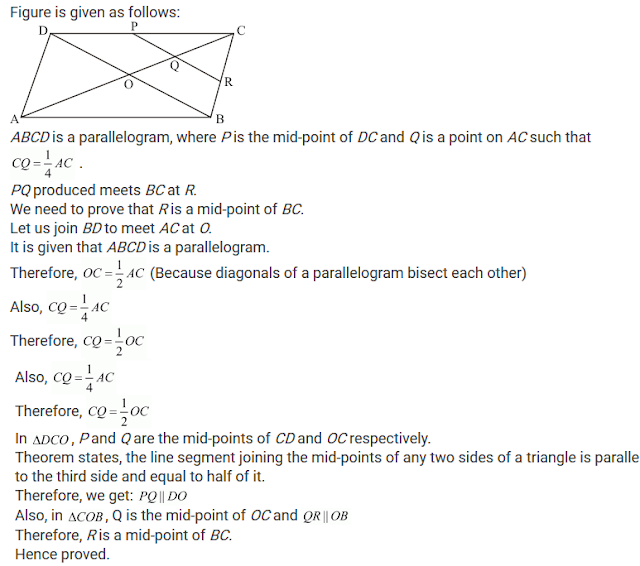16. In Fig. 14.100, ABCD and PQRC are rectangle and Q is the midpoint of AC. Prove that
(i) DP = PC
(ii) PR = 1/2 ∠AC

Solution17. ABCD is a parallelogram, E and F are the mid-points of AB and CD respectively. GH is any line intersecting AD, EF and BC at G, P and H respectively. Prove that GP = PH.

Solution18. RM and CN are perpendiculars to a line passing through the vertex A of a triangle ABC. IF L is the mid-point of BC, prove that LM = LN.

Solution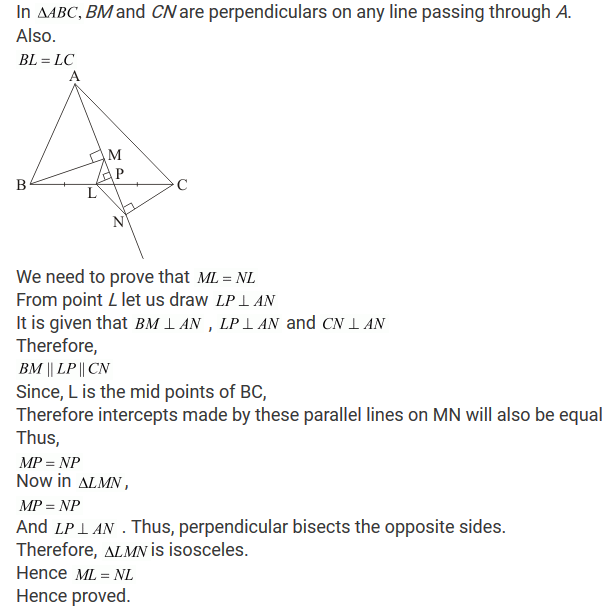19. Show that the line segments joining the mid-points of the opposite sides of a quadrilateral bisect each other.

Solution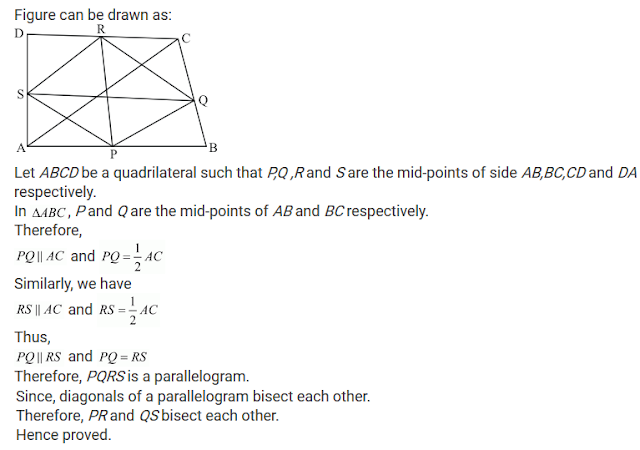20. Fill in the blanks to make the following statements correct:

(i) The triangle formed by joining the mid-points of the sides of an isosceles triangle is........
(ii) The triangle formed by joining the mid-points of the sides of a right triangle is ........
(iii) The figure formed by joining the mid-points of consecutive sides of a quadrilateral is ..........

Solution

(i) Isosceles

(ii) Right triangle
(iii) Parallelogram

X